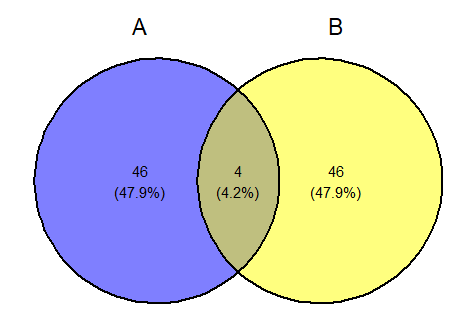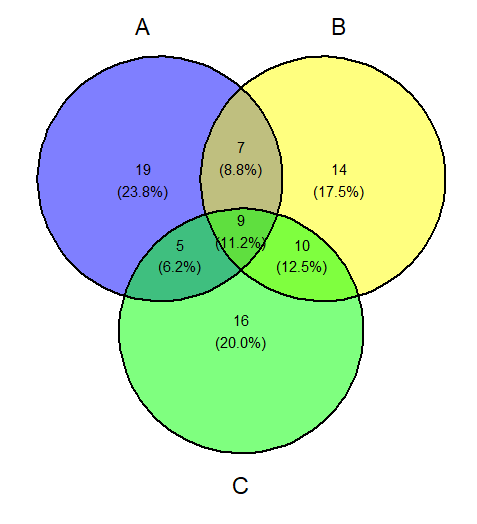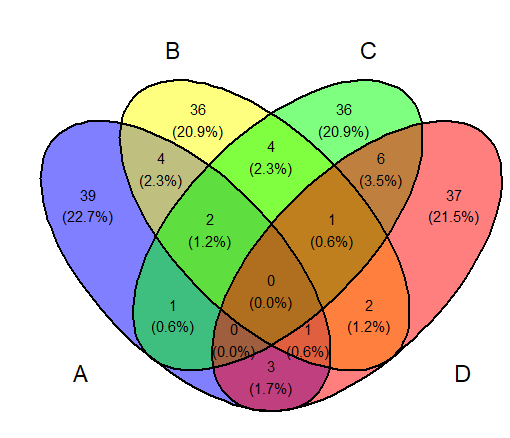Open in App
Not now

# ggvenn Package in R

• Last Updated : 02 Jun, 2022

In this article, we will be discussing the ggvenn package briefly with its working examples accordingly in the R programming language.

## ggvenn Package in R

The ggvenn package provides an easy-to-use way to draw Venn diagrams using the standard ggplot2 syntax and layout. The package hence makes it possible to match the design and style of Venn diagrams to other graphics created by the ggplot2 package.

## Draw Venn Diagram

In this approach to drawing the pairwise Venn diagram, the user needs to first install and import the ggvenn package to the working R console and then call the ggvenn() function from this package passed with the required parameter to get the pairwise Venn diagram accordingly in the R programming language.

### Syntax to install and import the ggvenn package:

```install.packages("ggvenn")
library("ggvenn")```

ggvenn() function: Plot Venn diagram with supports of a data frame or list as input as an independent function.

Syntax: ggvenn( data, columns)

Parameters:

• data: A dataframe or a list as input data.
• columns: A character vector use as an index to select columns/elements.

Example 1:

In this example, we have created the list of the 2 attributes, and then with all of the ggvenn() functions from the ggvenn package passed with the list name and the name of the attribute created we are getting the Pairwise Venn Diagram in R language.

## R

 `# Import required package ` `library``(``"ggvenn"``) ` ` `  `# Create Data ` `venn <- ``list``(A = ``sort``(``sample``(1:500, 50)), ` `                  ``B = ``sort``(``sample``(1:500, 50))) ` ` `  `# Create venn diagram Pairwise ` `ggvenn``(venn, ``c``(``"A"``, ``"B"``))`

Output:Example 2:

In this example, we have created the list of the 3 attributes, and then with all of the ggvenn() functions from the ggvenn package passed with the list name and the name of the attribute created we are getting the Venn Diagram of three set in R language.

## R

 `# Import required package ` `library``(``"ggvenn"``) ` ` `  `# Create Data ` `venn <-      ``list``(A = ``sort``(``sample``(1:100, 40)), ` `                  ``B = ``sort``(``sample``(1:100, 40)), ` `                  ``C = ``sort``(``sample``(1:100, 40))) ` ` `  `# Create venn diagram with three sets ` `ggvenn``(venn, ``c``(``"A"``, ``"B"``,``"C"``))`

Output:Example 3:

In this example, we have created the list of the 4 attributes, and then with all of the ggvenn() functions from the ggvenn package passed with the list name and the name of the attribute created we are getting the Venn Diagram of four set in R language.

## R

 `# Import required package ` `library``(``"ggvenn"``) ` ` `  `# Create Data ` `venn <-      ``list``(A = ``sort``(``sample``(1:500, 50)), ` `                  ``B = ``sort``(``sample``(1:500, 50)), ` `                  ``C = ``sort``(``sample``(1:500, 50)), ` `                  ``D = ``sort``(``sample``(1:500, 50))) ` ` `  `# Create venn diagram with three sets ` `ggvenn``(venn, ``c``(``"A"``, ``"B"``,``"C"``,``"D"``))`

Output:My Personal Notes arrow_drop_up
Related Articles